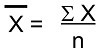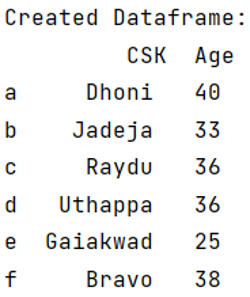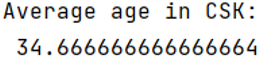# How to calculate average/mean of Pandas column?

Given a Pandas DataFrame, we have to calculate average or mean of column. By Pranit Sharma Last updated : September 21, 2023

Pandas is a special tool that allows us to perform complex manipulations of data effectively and efficiently. Inside pandas, we mostly deal with a dataset in the form of DataFrame. DataFrames are 2-dimensional data structures in pandas. DataFrames consist of rows, columns, and the data.

The most fascinating key point about pandas is that it contains numerous amounts of function to calculate almost everything mathematically and logically.

With the help of pandas, we can calculate the mean of any column in a DataFrame, the column values should be an integer or float values and not a string.

## Problem statement

Given a Pandas DataFrame, we have to calculate average or mean of column.

## What is mean?

Mean is nothing but an average value of a series of a number. Mathematically, the mean can be calculated as:Here, x̄ is the mean, x is the summation of all the values and n is the total number of values/elements.

Suppose we have a series of numbers from 1 to 10, then the average of this series will be:

```∑x = 1+2+3+4+5+6+7+8+9+10
∑x = 55
n = 10
x̄ = 55/10
x̄ = 5.5
```

## Calculating average/mean of Pandas column

In pandas, we use pandas.DataFrame['col'].mean() directly to calculate the average value of a column.

Note

To work with pandas, we need to import pandas package first, below is the syntax:

```import pandas as pd
```

Let us understand with the help of an example.

## Python program to calculate average/mean of Pandas column

```# Import pandas Package
import pandas as pd

# Creating dictionary
d = {
"Age": [40, 33, 36, 36, 25, 38],
}

# Creating a Dataframe
df = pd.DataFrame(d, index=["a", "b", "c", "d", "e", "f"])

print("Created Dataframe:\n", df, "\n")

# Calculating average age
result = df["Age"].mean()

# Display result
print("Average age in CSK:\n", result)
```

### Output

```Created Dataframe:
CSK  Age
a     Dhoni   40
c     Raydu   36
d   Uthappa   36
f     Bravo   38

Average age in CSK:
34.666666666666664
```